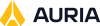# Algorithm Definitions

There are several schedule deconfliction algorithms that can be used to find a schedule solution. In all cases, the deconfliction algorithms will attempt to assign start times, durations, and resource assignments to each task.

To change the deconfliction algorithm, select Schedule -> Select Algorithm from the Menu Bar.

For a detailed discussion of the algorithms, see The Optwise Corporation Deconfliction Scheduler Algorithms (as used in STK Scheduler).

The available deconfliction algorithms are:

## Sequential Algorithm

The sequential algorithm is based on the One-Pass algorithm but uses the timeslot start time as its secondary task consideration sorting order (instead of timeslot desirability). This seemingly minor change makes a major improvement in solution quality for scheduling problems that include repetitive consumable resource depletion and replenishment. An example of this type of problem is satellite imagery collection planning where images are stored on-board a recorder and later downlinked to ground stations to free recorder space for additional collections.

## Multi-Pass Algorithm

The Multi-Pass algorithm uses the core deconfliction processing routines in the One-Pass algorithm, but in each of its multiple passes modifies the task order and the timeslot order prior to each assignment pass. A set of expert system rules is used to generate the possible task and time order lists. Each pass is graded based on a Figure Of Merit (FOM) and the best solution is returned. This algorithm will require more time than the One-Pass algorithm since it has to run through the One-Pass algorithm multiple times. The actual number of times it is run is based on the complexity of the problem. The multi-pass algorithm will usually produce better result than the one-pass algorithm.

## Neural Network Algorithm

The Neural algorithm uses a competition model in which all of the possible assignment possibilities compete to be assigned subject to the constraints just as many potential bidders compete for products at an auction. In this unique algorithm, the order of the task and timeslots are unimportant, but task priority and timeslot desirability affect the probability that a particular assignment will be chosen through the use of the Figure Of Merit. Since the algorithm does not bias the solution toward earliest time, a repair algorithm is run at the end to move tasks to the earliest possible time and then tests to see if any additional unassigned tasks can be added. Multiple runs can generate a family of solutions.

## Random Algorithm

The Random Algorithm searches for solutions in an unbiased manner to seed a random solution (and available options) and uses a repair algorithm to repair it. While the initial random solution will probably be invalid (in violation of constraints and having tasks with duration far less than desired) the repaired schedule will be valid. This algorithm's strength is that it is unbiased and can find solutions that cannot be predicted by rules. It is useful when a very unusual Figure Of Merit is used to grade the performance. While constraints are obeyed, they are not used to help fine the best solution.

## Squeaky-Wheel Algorithm

The concept behind a Squeaky-Wheel algorithm is straight forward. Multiple runs of a solver are done. After each run, tasks that are not assigned are noted and a credit is calculated that is used to bias the order in which candidate tasks (and task slots) are searched of subsequent runs. Tasks that are easy to assign will tend to fall later than tasks that are never assigned (Squeaky-Wheel tasks) as more and more runs are done. The Squeaky-Wheel algorithm uses the same basic search method as Random Algorithm. The difference is that after each run, instead of randomly choosing the slot order, the new slot order is biased by how many times the task has been missed.